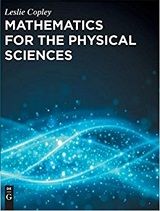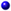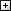Processing ......FreeComputerBooks.com Free Computer, Mathematics, Technical Books and Lecture Notes, etc.

Mathematics for the Physical Sciences
Want to know the elevation (AMSL) at any spot all over world? Click here for details.
• Title Mathematics for the Physical Sciences by Leslie Copley
• Author(s) Leslie Copley
• Publisher: Sciendo (December 15, 2014);
• Hardcover 446 pages
• eBook PDF Files
• Language: English
• ISBN-10/ASIN: 3110409453
• ISBN-13: 978-3110409451Book Description

This book provides a comprehensive introduction to the areas of mathematical physics. It combines all the essential math concepts into one compact, clearly written reference and illustrates the mathematics with numerous physical examples drawn from contemporary research.

It begins with a thorough introduction to complex analysis, which is then used to understand the properties of ordinary differential equations and their solutions. The latter are obtained in both series and integral representations. Integral transforms are introduced, providing an opportunity to complement complex analysis with techniques that flow from an algebraic approach.

This moves naturally into a discussion of eigenvalue and boundary vale problems. A thorough discussion of multi-dimensional boundary value problems then introduces the reader to the fundamental partial differential equations and special functions of mathematical physics. Moving to non-homogeneous boundary value problems the reader is presented with an analysis of Green's functions from both analytical and algebraic points of view. This leads to a concluding chapter on integral equations.

Reviews, Ratings, and Recommendations: Related Book Categories: Read and Download Links:Similar Books:All CategoriesRecent BooksIT Research LibraryMiscellaneous BooksComputer LanguagesComputer ScienceData Science/DatabasesElectronic EngineeringJava and Java EE (J2EE)Linux and UnixMathematicsMicrosoft and .NETMobile ComputingNetworking and CommunicationsSoftware EngineeringSpecial TopicsWeb Programming